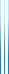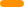# PublicationsPapers

## Optimal sets of questions for Twenty Questions

Yuval Filmus and Idan Mehalel
Submitted

In the Twenty Questions game, Bob picks a number $x$ according to a distribution $\mu$, and Alice, who known $\mu$, attempts to find $x$ by asking Bob Yes/No questions, which Bob answers truthfully. Alice’s goal is to minimize the expected number of questions. The optimal strategy for the game is given by a Huffman code.

A set of questions is optimal if using only questions from this set, for every $\mu$ there exists a strategy for Alice with the optimal expected number of questions. In previous work, we have shown that for every $n$ there is an optimal set of questions of size roughly $1.25^n$, and moreover, this is optimal for infinitely many $n$ (up to subexponential factors), namely, $n \approx 1.25 \cdot 2^m$.

In this paper, we prove that the optimal number of questions for $n$ of the form $\beta \cdot 2^m$ (where $\beta \in [1,2)$ is $2^{-G(\beta)n}$ (up to subexponential factors), where $G(\beta)$ is an explicit function which is strictly larger than $-\log_2 1.25$ for all $\beta \neq 1.25$.

We also extend the entire setup to $d$-ary questions, showing that the analog of the magic constant $1.25$ in this case is $1 + (d-1)/d^{d/(d-1)}$.

## BibTeX

@misc{FilmusMehalel2021,
author = {Yuval Filmus and Idan Mehalel},
title = {Optimal sets of questions for {T}wenty {Q}uestions},
year = {2021}
}copy to clipboard